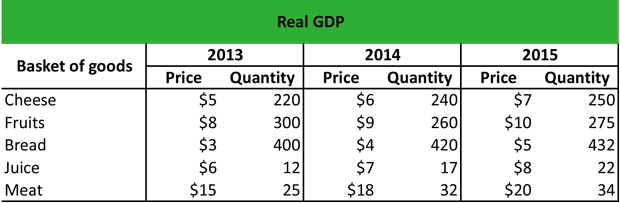# What is Real GDP?

Definition: Real GDP, also known as inflation-adjusted gross domestic product, measures the value of finished goods and services at constant base-year prices. The real gross domestic product is adjusted for inflation or deflation with the use of nominal GDP and the GDP deflator.

## What Does Real GDP Mean?

What is the definition of real GPD? This includes changes in the general price level in a given year to provide an accurate picture of an economy’s growth using base-year prices. If the general price level changes from one year to the next, it is difficult to compare the amount of output across different years. By valuing the entire output of an economy using the average price of a base year, economists can use this measurement to analyze an economy’s purchasing power and growth potential in the long-term.

The real GDP formula includes consumption, investment, public expenditure and net exports and is usually lower than the nominal GDP that includes inflation. In fact, the real GDP reflects the nominal GDP of an economy if there were no prices changes due to inflation.

Let’s look at an example.

## Example

Taylor constructs a basket of goods to calculate the real GDP on certain finished goods.First, he calculates the nominal GPD equation for each goods as follows:

Cheese: (\$5 x 220) + (\$6 x 240) + (\$7 x 250) = \$4,290
Fruits: (\$8 x 300) + (\$9 x 260) + (\$10 x 275) = \$7,490
Bread: (\$3 x 400) + (\$4 x 420) + (\$5 x 432) = \$5,040
Juice: (\$6 x 12) + (\$7 x 17) + (\$8 x 22) = \$367
Meat: (\$15 x 25) + (\$18 x 32) + (\$20 x 34) = \$1,631

Then, he calculates the real GPD equation using 2013 as base year:

Cheese: (\$5 x 220) + (\$5 x 240) + (\$5 x 250) = \$3,550
Fruits: (\$8 x 300) + (\$8 x 260) + (\$8 x 275) = \$6,680
Bread: (\$3 x 400) + (\$3 x 420) + (\$3 x 432) = \$3,756
Juice: (\$6 x 12) + (\$6 x 17) + (\$6 x 22) = \$306
Meat: (\$15 x 25) + (\$15 x 32) + (\$15 x 34) = \$1,365

The real GDP is lower than the nominal GDP because the nominal GDP includes inflation. If Taylor wants to calculate the GDP deflator he will divide the nominal GDP by the real GDP as follows:

Cheese: \$4,290 / \$3,550 x 100 = \$121
Fruits: \$7,490 / \$6,680 x 100 = \$112
Bread: \$5,040 / \$3,756 x 100 = \$134
Juice: \$367 / \$306 x 100 = \$120
Meat: \$1,631 / \$1,365 x 100 = \$119

## Summary Definition

Define Real GDP: Real gross domestic product is the value of goods and services produced by a country for a given year adjusted for price changes like inflation.